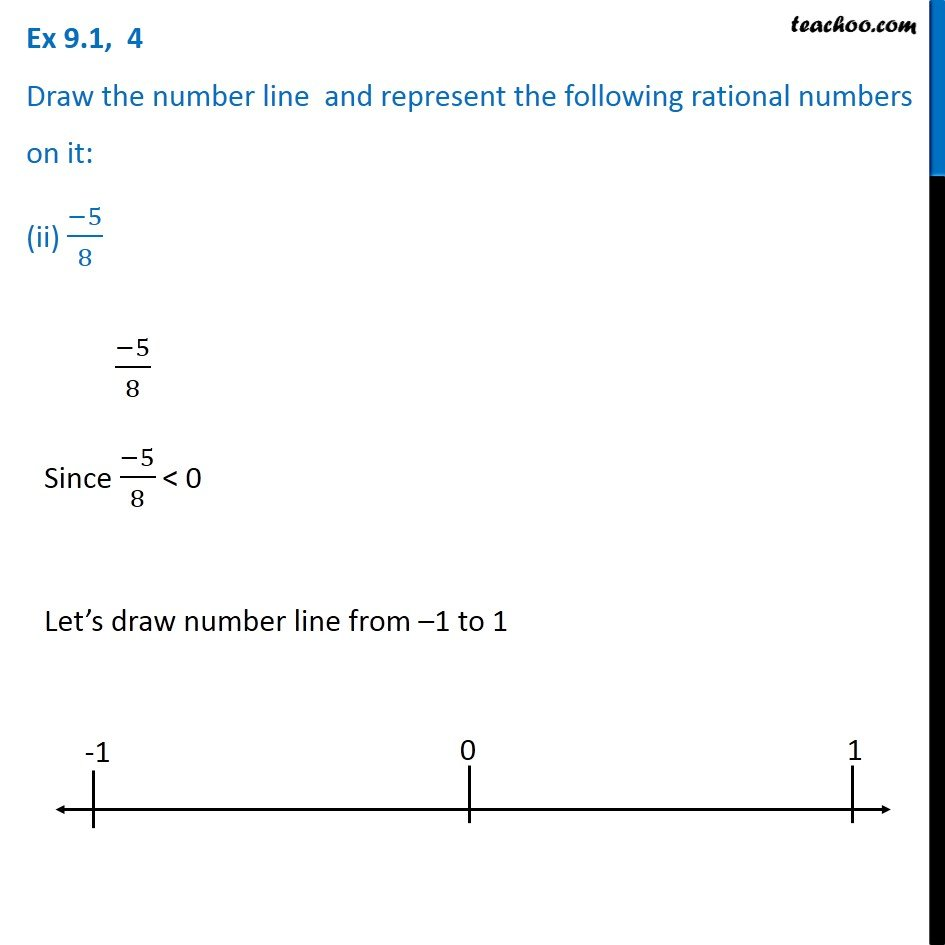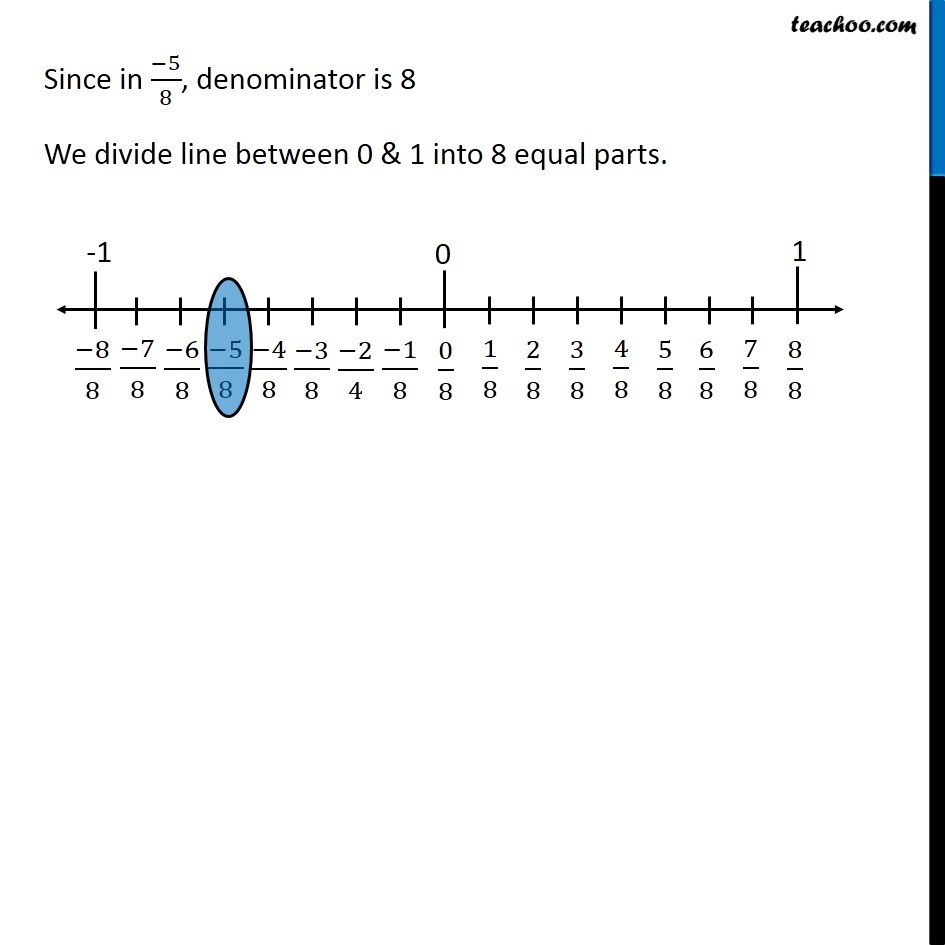Ex 9.1

Chapter 9 Class 7 Rational Numbers
Serial order wiseGet live Maths 1-on-1 Classs - Class 6 to 12

### Transcript

Ex 9.1, 4 Draw the number line and represent the following rational numbers on it: (ii) (−5)/8 (−5)/8 Since (−5)/8 < 0 Let’s draw number line from –1 to 1 Since in (−5)/8, denominator is 8 We divide line between 0 & 1 into 8 equal parts.